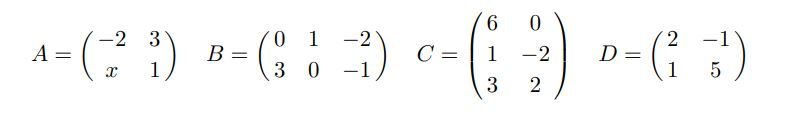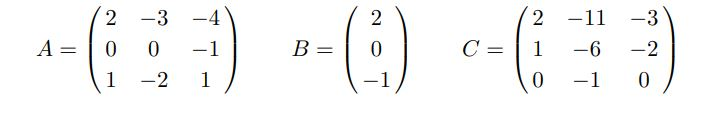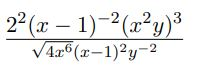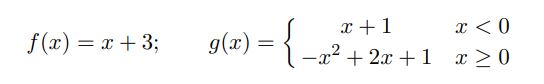# OMAS110: Show by Calculating the Product AC that C is the Inverse Matrix of A: Fundamentals of Mathematics Assignment, OU, Australia

 Subject OMAS110: Fundamentals of Mathematics

Please show all your work. Where possible give the exact answer.

1. Consider the following matricesWhere possible, find the following. If not possible give the reason.
(a) AB
(b) DA
(c) B + C
(d) AC
(e) 2D + BC
(f) The value of x for which A does not have an inverse.

2. Consider the three matrices(a) Show by calculating the product AC that C is the inverse matrix of A
(b) Hence calculate the solution to the linear system AX = B.

3. Simplify4. Solve the following inequality problems.

You must give your answer using interval notation. You must also give you an answer in graphical form (using appropriate shading on the number line).

(a) 4 − x ≤ −2 or x + 3 > 3x − 1

(b) 1 − 2x < 7 and 3x − 2 ≤ 1.

5. Evaluate the following

(a) |3 − 6| − |4 − 9|
(b) ||1 + 4| − |3 − 7||

6. Let f(x) and g(x) be defined by(a) Evaluate (f + g)(−2), (f − g)(−1), (fg)(1) and (f /g)(2).
(b) Find all x for which f(x) = g(x). You must give the exact answer and
show all working.

## Get Help By Expert

Our assignment helpers are always ready to give the Open University (OU) students in Australia math assignment help at any time when they need it. Our online assignment expert emphasizes the structure of the (OMAS110) Fundamentals of Mathematics assignment through analyzing the content or topic step by step.